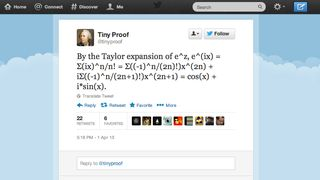# Mathematically beyond-us Twitter account will make you feel stupid on a daily basisWe totally get it

It's all gone a bit Good Will Hunting over on Twitter where an equation-proving account is the novelty order of the day.

Whoever's behind @TinyProof is making us feel cerebrally-challenged with its 140-character equation solving ways.

The account sets out to prove complicated mathematical equations for your tweeting pleasure, and includes such gems as, "Assume f : A -> P(A) is onto, and let B = { x ∈ A | x ∉ f(x) }. By assumption, there is an x ∈ A such that f(x) = B. Then x ∈ B iff x ∉ B."

Which is exactly what we were about to tweet. Enjoy.